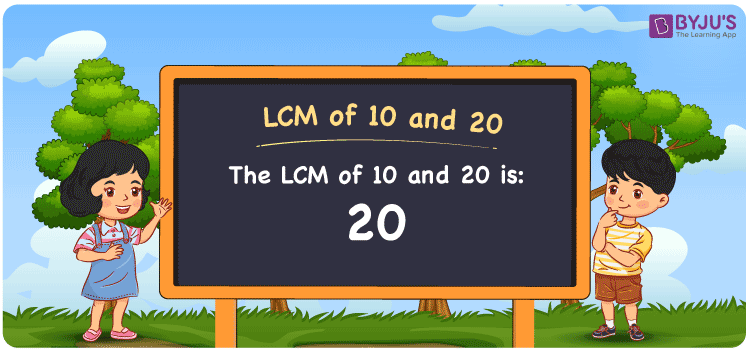Checkout JEE MAINS 2022 Question Paper Analysis : Checkout JEE MAINS 2022 Question Paper Analysis :

# LCM of 10 and 20

LCM of 10 and 20 is 20. Students can obtain the Least common multiple of 10 and 20 from the common multiples. (10, 20, 30, 40, 50, 60, 70, ….) and (20, 40, 60, 80, 100, ,….) are the multiples of 10 and 20. The methods used to find the LCM of two numbers are prime factorisation, division and by listing the multiples.

## What is LCM of 10 and 20?

The answer to this question is 20. The LCM of 10 and 20 using various methods is shown in this article for your reference. The LCM of two non-zero integers, 10 and 20, is the smallest positive integer 20 which is divisible by both 10 and 20 with no remainder.## How to Find LCM of 10 and 20?

LCM of 10 and 20 can be found using three methods:

• Prime Factorisation
• Division method
• Listing the multiples

### LCM of 10 and 20 Using Prime Factorisation Method

The prime factorisation of 10 and 20, respectively, is given by:

10 = 2 x 5 = 2¹ x 5¹

20 = 2 x 2 x 5 = 2² x 5¹

LCM (10, 20) = 20

### LCM of 10 and 20 Using Division Method

We’ll divide the numbers (10, 20) by their prime factors to get the LCM of 10 and 20 using the division method (preferably common). The LCM of 10 and 20 is calculated by multiplying these divisors.

 2 10 20 2 5 10 5 5 5 x 1 1

No further division can be done.

Hence, LCM (10, 20) = 20

### LCM of 10 and 20 Using Listing the Multiples

To calculate the LCM of 10 and 20 by listing out the common multiples, list the multiples as shown below.

 Multiples of 10 Multiples of 20 10 20 20 40 30 60 40 80 50 100

LCM (10, 20) = 20

## Video Lesson on Applications of LCM## LCM of 10 and 20 Solved Examples

Find the smallest number that is divisible by 10 and 20 exactly.

Solution:

We know that;

The smallest number which is exactly divisible by 10 and 20 is the LCM.

Multiples of 10 = 10, 20, 30, 40, 50, 60, 70, …..

Multiples of 20 = 20, 40, 60, 80, 100, 120, ….

Hence, the LCM of 10 and 20 is 20.

## Frequently Asked Questions on LCM of 10 and 20

### In the numbers 11, 20, 2 and 32, find the LCM of 10 and 20.

The LCM value is the smallest common multiple evenly divisible by 10 and 20. The number which satisfies this condition is 20.

### Determine the GCF if the LCM of 10 and 20 is 20.

LCM x GCF = 10 x 20

Given

LCM of 10 and 20 = 20

20 x GCF = 200

GCF = 200/20 = 10

### Show the relation between GCF and LCM of 10 and 20.

The relation between GCF and LCM of 10 and 20 is:

LCM x GCF = 10 x 20

LCM x GCF = 200